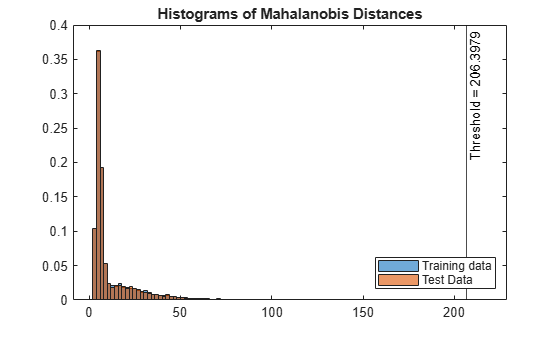## Unsupervised Anomaly Detection

This topic introduces the unsupervised anomaly detection features for multivariate sample data available in Statistics and Machine Learning Toolbox™, and describes the workflows of the features for outlier detection (detecting anomalies in training data) and novelty detection (detecting anomalies in new data with uncontaminated training data).

For unlabeled multivariate sample data, you can detect anomalies by using isolation forest, one-class support vector machine (OCSVM), and Mahalanobis distance. These methods detect outliers either by training a model or by learning parameters. For novelty detection, you train a model or learn parameters with uncontaminated training data (data with no outliers) and detect anomalies in new data by using the trained model or learned parameters.

• Isolation forest — The Isolation Forest algorithm detects anomalies by isolating them from normal points using an ensemble of isolation trees. Detect outliers by using the `iforest` function, and detect novelties by using the object function `isanomaly`.

• One-class support vector machine (OCSVM) — One-Class Learning, or unsupervised SVM, tries to separate data from the origin in the transformed high-dimensional predictor space. Train an OCSVM model by using the `fitcsvm` function, and then detect outliers and novelties by using the `resubPredict` and `predict` object functions, respectively.

• Mahalanobis Distance — If sample data follows a multivariate normal distribution, then the squared Mahalanobis distances from samples to the distribution follow a chi-square distribution. Therefore, you can use the distances to detect anomalies based on the critical values of the chi-square distribution. For outlier detection, use the `robustcov` function to compute robust Mahalanobis distances. For novelty detection, you can compute Mahalanobis distances by using the `robustcov` and `pdist2` functions.

### Outlier Detection

This example illustrates the workflows of the three unsupervised anomaly detection methods (isolation forest, OCSVM, and Mahalanobis distance) for outlier detection.

Load the `humanactivity` data set, which contains the variables `feat` and `actid`. The variable `feat` contains the predictor data matrix of 60 features for 24,075 observations, and the response variable `actid` contains the activity IDs for the observations as integers. This example uses the `feat` variable for anomaly detection.

`load humanactivity`

Find the size of the variable `feat`.

`[N,D] = size(feat)`
```N = 24075 ```
```D = 60 ```

Assume that the fraction of outliers in the data is 0.05.

`contaminationFraction = 0.05;`

Isolation Forest

Detect outliers by using the `iforest` function.

Train an isolation forest model by using the `iforest` function. Specify the fraction of outliers (`ContaminationFraction`) as 0.05.

```rng("default") % For reproducibility [forest,tf_forest,s_forest] = iforest(feat, ... ContaminationFraction=contaminationFraction);```

`forest` is an `IsolationForest` object. `iforest` also returns the anomaly indicators (`tf_forest`) and anomaly scores (`s_forest`) for the data (`feat`). `iforest` determines the score threshold value (`forest.ScoreThreshold`) so that the function detects the specified fraction of observations as outliers.

Plot a histogram of the score values. Create a vertical line at the score threshold corresponding to the specified fraction.

```figure histogram(s_forest,Normalization="probability") xline(forest.ScoreThreshold,"k-", ... join(["Threshold =" forest.ScoreThreshold])) title("Histogram of Anomaly Scores for Isolation Forest")```Check the fraction of detected anomalies in the data.

`OF_forest = sum(tf_forest)/N`
```OF_forest = 0.0496 ```

The outlier fraction can be smaller than the specified fraction (0.05) when the scores can have tied values at the threshold.

One-Class Support Vector Machine (OCSVM)

Train an OCSVM model by using the `fitcsvm` function, and then detect outliers by using the `resubPredict` function.

Train a support vector machine model for one-class learning by using the `fitcsvm` function. The function trains a model for one-class learning if the class label variable is a vector of ones. Specify the fraction of outliers (`OutlierFraction`) as 0.05.

```Mdl = fitcsvm(feat,ones(size(feat,1),1), ... OutlierFraction=contaminationFraction, ... KernelScale="auto",Standardize=true);```

`Mdl` is a `ClassificationSVM` object.

Compute the outlier scores for `feat` by using the `resubPredict` function.

`[~,s_OCSVM] = resubPredict(Mdl);`

Negative score values indicate that the corresponding observations are outliers. Obtain the anomaly indicators.

`tf_OCSVM = s_OCSVM < 0;`

Plot a histogram of the score values.

```figure histogram(s_OCSVM,Normalization="probability"); xline(0,"k-","Threshold = 0") title("Histogram of Anomaly Scores for OCSVM")```Check the fraction of detected anomalies in the data.

`OF_OCSVM = sum(tf_OCSVM)/N`
```OF_OCSVM = 0.0500 ```

`fitcsvm` trains the bias term of the SVM model so that the specified fraction of the training observations has negative scores. Therefore, `OF_OCSVM` is close to the specified fraction value.

Mahalanobis Distance

Use the `robustcov` function to compute robust Mahalanobis distances and robust estimates for the mean and covariance of the data.

Compute the Mahalanobis distance from `feat` to the distribution of `feat` by using the `robustcov` function. Specify the fraction of outliers (`OutlierFraction`) as 0.05. `robustcov` minimizes the covariance determinant over 95% of the observations.

```[sigma,mu,s_robustcov,tf_robustcov_default] = robustcov(feat, ... OutlierFraction=contaminationFraction);```

`robustcov` finds the robust covariance matrix estimate (`sigma`) and robust mean estimate (`mu`), which are less sensitive to outliers than the estimates from the `cov` and `mean` functions. The `robustcov` function also computes the Mahalanobis distances (`s_robustcov`) and the outlier indicators (`tf_robustcov_default`). By default, the function assumes that the data set follows a multivariate normal distribution, and identifies 2.5% of input observations as outliers based on the critical values of the chi-square distribution.

If the data set satisfies the normality assumption, then the squared Mahalanobis distance follows a chi-square distribution with `D` degrees of freedom, where `D` is the dimension of the data. In that case, you can find a new threshold by using the `chi2inv` function to detect the specified fraction of observations as outliers.

```s_robustcov_threshold = sqrt(chi2inv(1-contaminationFraction,D)); tf_robustcov = s_robustcov > s_robustcov_threshold;```

Create a distance-distance plot (DD plot) to check the multivariate normality of the data.

```figure d_classical = pdist2(feat,mean(feat),"mahalanobis"); gscatter(d_classical,s_robustcov,tf_robustcov,"kr",".x") xline(s_robustcov_threshold,"k-") yline(s_robustcov_threshold,"k-", ... join(["Threshold = " s_robustcov_threshold])); l = refline([1 0]); l.Color = "k"; xlabel("Mahalanobis Distance") ylabel("Robust Distance") legend("Normal Points","Outliers",Location="northwest") title("Distance-Distance Plot")```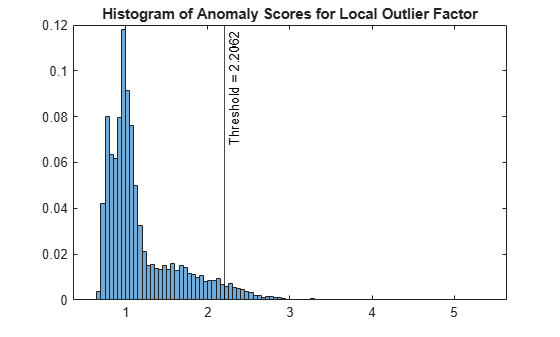Zoom in the axes to see the normal points.

```xlim([0 10]) ylim([0 10])```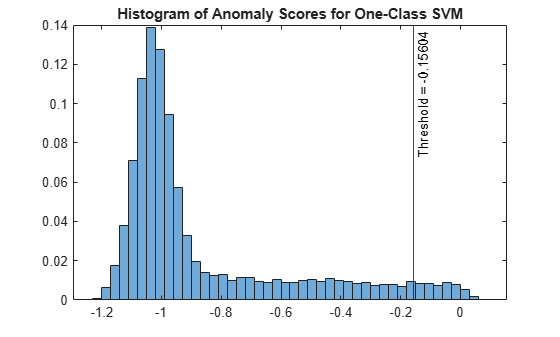If a data set follows a multivariate normal distribution, then data points cluster tightly around the 45 degree reference line. The DD plot indicates that the data set does not follow a multivariate normal distribution.

Because the data set does not satisfy the normality assumption, use the quantile of the distance values for the cumulative probability (`1 — contaminationFraction`) to find a threshold.

`s_robustcov_threshold = quantile(s_robustcov,1-contaminationFraction);`

Obtain the anomaly indicators for `feat` using the new threshold `s_robustcov_threshold`.

`tf_robustcov = s_robustcov > s_robustcov_threshold;`

Check the fraction of detected anomalies in the data.

`OF_robustcov = sum(tf_robustcov)/N`
```OF_robustcov = 0.0500 ```

Compare Detected Outliers

To visualize the detected outliers, reduce the data dimension by using the `tsne` function.

```rng("default") % For reproducibility T = tsne(feat,Standardize=true,Perplexity=100,Exaggeration=20);```

Plot the normal points and outliers in the reduced dimension. Compare the results of the three methods: the isolation forest algorithm, OCSVM model, and robust Mahalanobis distance from `robustcov`.

```figure tiledlayout(2,2) nexttile gscatter(T(:,1),T(:,2),tf_forest,"kr",".x",[],"off") title("Isolation Forest") nexttile(3) gscatter(T(:,1),T(:,2),tf_OCSVM,"kr",".x",[],"off") title("OCSVM") nexttile(4) gscatter(T(:,1),T(:,2),tf_robustcov,"kr",".x",[],"off") title("Robust Mahalanobis Distance") l = legend("Normal Points","Novelties"); l.Layout.Tile = 2;```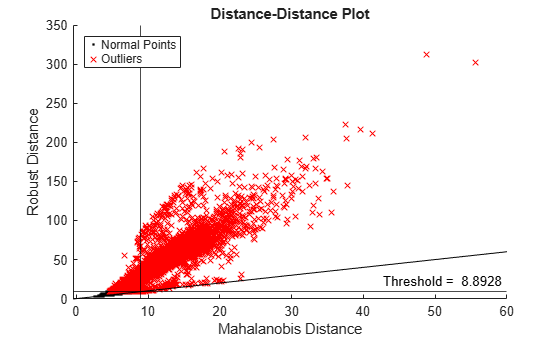The novelties identified by the three methods are located near each other in the reduced dimension. Compute the fraction of outliers that the three methods have in common.

`sum(tf_forest.*tf_OCSVM.*tf_robustcov)/N`
```ans = 0.0298 ```

The three methods identify about 3% of the data (`feat`) as outliers.

You can also visualize observation values using the two most important features selected by the `fsulaplacian` function.

```idx = fsulaplacian(feat); figure t = tiledlayout(2,2); nexttile gscatter(feat(:,idx(1)),feat(:,idx(2)),tf_forest,"kr",".x",[],"off") title("Isolation Forest") nexttile(3) gscatter(feat(:,idx(1)),feat(:,idx(2)),tf_OCSVM,"kr",".x",[],"off") title("OCSVM") nexttile(4) gscatter(feat(:,idx(1)),feat(:,idx(2)),tf_robustcov,"kr",".x",[],"off") title("Mahalanobis Distance") l = legend("Normal Points","Novelties"); l.Layout.Tile = 2; xlabel(t,join(["Column" idx(1)])) ylabel(t,join(["Column" idx(2)]))```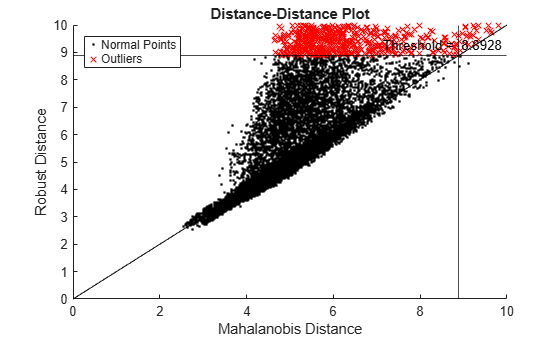### Novelty Detection

This example illustrates the workflows of the three unsupervised anomaly detection methods (isolation forest, OCSVM, and Mahalanobis distance) for novelty detection.

Load the `humanactivity` data set, which contains the variables `feat` and `actid`. The variable `feat` contains the predictor data matrix of 60 features for 24,075 observations, and the response variable `actid` contains the activity IDs for the observations as integers. This example uses the `feat` variable for anomaly detection.

`load humanactivity`

Partition the data into training and test sets by using the `cvpartition` function. Use 50% of the observations as training data and 50% of the observations as test data for novelty detection.

```rng("default") % For reproducibility c = cvpartition(actid,Holdout=0.50); trainingIndices = training(c); % Indices for the training set testIndices = test(c); % Indices for the test set XTrain = feat(trainingIndices,:); XTest = feat(testIndices,:);```

Assume that the training data is not contaminated (no outliers).

Find the size of the training and test sets.

`[N,D] = size(XTrain)`
```N = 12038 ```
```D = 60 ```
`NTest = size(XTest,1)`
```NTest = 12037 ```

Isolation Forest

Detect novelties using the object function `isanomaly` after training an isolation forest model by using the `iforest` function.

Train an isolation forest model.

`[forest,tf_forest,s_forest] = iforest(XTrain);`

`forest` is an `IsolationForest` object. `iforest` also returns the anomaly indicators (`tf_forest`) and anomaly scores (`s_forest`) for the training data (`XTrain`). By default, `iforest` treats all training observations as normal observations, and sets the score threshold (`forest.ScoreThreshold`) to the maximum score value.

Use the trained isolation forest model and the object function `isanomaly` to find novelties in `XTest`. The `isanomaly` function identifies observations with scores above the threshold (`forest.ScoreThreshold`) as novelties.

`[tfTest_forest,sTest_forest] = isanomaly(forest,XTest);`

The `isanomaly` function returns the anomaly indicators (`tfTest_forest`) and anomaly scores (`sTest_forest`) for the new data.

Plot histograms of the score values. Create a vertical line at the score threshold.

```figure histogram(s_forest,Normalization="probability") hold on histogram(sTest_forest,Normalization="probability") xline(forest.ScoreThreshold,"k-", ... join(["Threshold =" forest.ScoreThreshold])) legend("Training data","New data",Location="southeast") title("Histograms of Anomaly Scores for Isolation Forest") hold off```The anomaly score distribution of the test data is similar to that of the training data, so `isanomaly` detects a small number of anomalies in the test data.

Check the fraction of detected anomalies in the test data.

`NF_forest = sum(tfTest_forest)/NTest`
```NF_forest = 8.3077e-05 ```

Display the observation index of the anomalies in the test data.

`idx_forest = find(tfTest_forest)`
```idx_forest = 3422 ```

One-Class Support Vector Machine (OCSVM)

Train an OCSVM model by using the `fitcsvm` function, and then detect anomalies in new data by using the `predict` object function.

Train a support vector machine model for one-class learning by using the `fitcsvm` function. The function trains a model for one-class learning if the class label variable is a vector of ones. Specify the fraction of outliers (`OutlierFraction`) as 0.

```Mdl = fitcsvm(XTrain,ones(size(XTrain,1),1),OutlierFraction=0, ... KernelScale="auto",Standardize=true);```

`Mdl` is a `ClassificationSVM` object.

Compute the outlier scores for `XTrain` by using the `resubPredict` function.

`[~,s_OCSVM] = resubPredict(Mdl);`

Compute the novelty scores for `XTest` by using the `predict` function.

`[~,sTest_OCSVM] = predict(Mdl,XTest);`

Negative score values indicate that the corresponding observations are anomalies. Obtain the anomaly indicators.

`tfTest_OCSVM = sTest_OCSVM < 0;`

Plot histograms of the score values.

```figure histogram(s_OCSVM,Normalization="probability"); hold on histogram(sTest_OCSVM,Normalization="probability"); xline(0,"k-","Threshold = 0") legend("Training data","New Data",Location="best") title("Histograms of Anomaly Scores for OCSVM") hold off```Check the fraction of detected anomalies in the test data.

`NF_OCSVM = sum(tfTest_OCSVM)/NTest`
```NF_OCSVM = 0.0707 ```

Display the observation index of the anomalies in the test data.

`idx_OCSVM = find(tfTest_OCSVM)`
```idx_OCSVM = 851×1 1061 1443 1444 1445 1447 1448 1449 1450 1451 1453 ⋮ ```

Mahalanobis Distance

Use the `robustcov` function to compute Mahalanobis distances of training data, and use the `pdist2` function to compute Mahalanobis distances of new data.

Compute the Mahalanobis distance from `XTrain` to the distribution of `XTrain` by using the `robustcov` function. Specify the fraction of outliers (`OutlierFraction`) as 0.

`[sigma,mu,s_mahal] = robustcov(XTrain,OutlierFraction=0);`

`robustcov` also returns the estimates of covariance matrix (`sigma`) and mean (`mu`), which you can use to compute distances of new data.

Use the maximum value of `s_mahal` as the score threshold for novelty detection.

`s_mahal_threshold = max(s_mahal);`

Compute the Mahalanobis distance from `XTest` to the distribution of `XTrain` by using the `pdist2` function.

`sTest_mahal = pdist2(XTest,mu,"mahalanobis",sigma);`

Obtain the anomaly indicators for `XTest`.

`tfTest_mahal = sTest_mahal > s_mahal_threshold;`

Plot histograms of the score values.

```figure histogram(s_mahal,Normalization="probability"); hold on histogram(sTest_mahal,Normalization="probability"); xline(s_mahal_threshold,"k-", ... join(["Threshold =" s_mahal_threshold])) legend("Training data","New Data",Location="southeast") title("Histograms of Mahalanobis Distances") hold off```Check the fraction of detected anomalies in the test data.

`NF_mahal = sum(tfTest_mahal)/NTest`
```NF_mahal = 8.3077e-05 ```

Display the observation index of the anomalies in the test data.

`idx_mahal = find(tfTest_mahal)`
```idx_mahal = 3654 ```

Compare Detected Anomalies

To visualize the detected anomalies, reduce the data dimension by using the `tsne` function.

```rng("default") % For reproducibility T = tsne(feat,Standardize=true,Perplexity=100,Exaggeration=20); XTest2D = T(testIndices,:);```

Plot the normal points and novelties in the reduced dimension. Compare the results of the three methods: the isolation forest algorithm, OCSVM model, and Mahalanobis distance from `mahal`.

```figure tiledlayout(2,2) nexttile gscatter(XTest2D(:,1),XTest2D(:,2),tfTest_forest,"kr",".x",[],"off") title("Isolation Forest") nexttile(3) gscatter(XTest2D(:,1),XTest2D(:,2),tfTest_OCSVM,"kr",".x",[],"off") title("OCSVM") nexttile(4) gscatter(XTest2D(:,1),XTest2D(:,2),tfTest_mahal,"kr",".x",[],"off") title("Mahalanobis Distance") l = legend("Normal Points","Novelties"); l.Layout.Tile = 2;```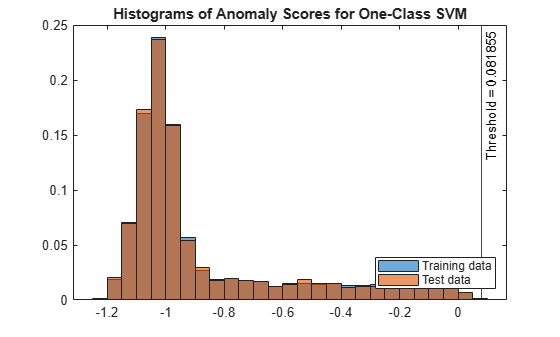The novelty identified by the isolation forest algorithm (`idx_forest`) and the novelty identified by the Mahalanobis distance (`idx_mahal`) are different, but they are located near each other in the reduced dimension.

Check if the novelties identified by OCSVM contain the novelties identified by the isolation forest algorithm and Mahalanobis distance.

`ismember(idx_forest,idx_OCSVM)`
```ans = logical 1 ```
`ismember(idx_mahal,idx_OCSVM)`
```ans = logical 1 ```

You can also visualize observation values using the two most important features selected by the `fsulaplacian` function.

```idx = fsulaplacian([XTrain; XTest]); figure t = tiledlayout(2,2); nexttile gscatter(XTest(:,idx(1)),XTest(:,idx(2)),tfTest_forest,"kr",".x",[],"off") title("Isolation Forest") nexttile(3) gscatter(XTest(:,idx(1)),XTest(:,idx(2)),tfTest_OCSVM,"kr",".x",[],"off") title("OCSVM") nexttile(4) gscatter(XTest(:,idx(1)),XTest(:,idx(2)),tfTest_mahal,"kr",".x",[],"off") title("Mahalanobis Distance") l = legend("Normal Points","Novelties"); l.Layout.Tile = 2; xlabel(t,join(["Column" idx(1)])) ylabel(t,join(["Column" idx(2)]))```